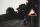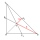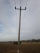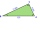# Planimetrics - math word problems

Study plane measurements, including angles, distances, and areas. In other words - measurement and calculation of shapes in the plane. Perimeter and area of plane shapes.

#### Number of problems found: 1947

• DodecagonCalculate the size of the smaller of the angles determined by lines A1 A4 and A2 A10 in the regular dodecagon A1A2A3. .. A12. Express the result in degrees.
• Regular prismThe regular four-sided prism has a base of 25 cm2 and a surface of 210 cm2. Find its volume.5 km long road begins at an altitude 500 meters above sea level and ends at a altitude 521 ASL. How many permille road rises?
• TilesThe room has dimensions 12 m and 5.6 m. Determine the number of square tiles and their largest possible size to cover them room's floor.
• Medians 2:1Median to side b (tb) in triangle ABC is 12 cm long. a. What is the distance of the center of gravity T from the vertex B? b, Find the distance between T and the side b.
• Right isosceles triangleRight isosceles triangle has an altitude x drawn from the right angle to the hypotenuse dividing it into 2 equal segments. The length of one segment is 5 cm. What is the area of the triangle?
• Concrete blockDetermine the volume of concrete block whose one edge of the base has a length 3 meters, body diagonal is 13 meters and its height is 12 meters.
• Rotary coneRotary cone whose height is equal to the circumference of the base, has a volume 229 cm3. Calculate the radius of the base circle and height of the cone.
• Telegraph polesThe bases of two adjacent telegraph poles have a height difference of 10.5 m. How long do the wires connect the two poles if the slope is 39° 30´?
• Rhombus and diagonalsThe a rhombus area is 150 cm2 and the ratio of the diagonals is 3:4. Calculate the length of its height.
• SimilarityAre two right triangles similar to each other if the first one has an acute angle 70°, and the second one has an acute angle 20°?
• Photo frameA square shape photo with a side length of 20 cm is framed by a 4 cm wide bar. Find the external frame size of this photo (the frame is from all sides)
• Percent changeIf the length of a rectangle is increased by 25% and the width is decreased by 10%, the area of the rectangle is larger than the area of the original rectangle by what percent?
• Triangle and its heightsCalculate the length of the sides of the triangle ABC, if va=5 cm, vb=7 cm and side b is 5 cm shorter than side a.
• EmbankmentThe railway embankment 300 m long has a cross section of an isosceles trapezoid with bases of 14 m and 8 m. The trapezoidal arms are 5 m long. Calculate how much m3 of soil is in the embankment?
• Square metal sheetFour squares of 300 mm side were cut out from a square sheet metal plate with a side of 0,7 m. Express the fraction and the percentage of waste from the square metal sheet.
• Hexagonal pyramidPlease calculate the height of a regular hexagonal pyramid with a base edge of 5cm and a wall height of w = 20cm. Please sketch a picture.
• RectangleDraw a rectangle with the sides a = 4 cm, b = 5 cm. Mark the center of symmetry S and all axes of symmetry. How many axes of symmetry has? Write down.
• ThereThere is a triangle ABC: A (-2,3), B (4, -1), C (2,5). Determine the general equations of the lines on which they lie: a) AB side, b) height to side c, c) Axis of the AB side, d) median ta to side a
• TrianglesEquilateral triangle with side 40 cm has the same perimeter as an isosceles triangle with arm of 45 cm. Calculate the base x of an isosceles triangle.

Do you have an interesting mathematical word problem that you can't solve it? Submit a math problem, and we can try to solve it.

We will send a solution to your e-mail address. Solved examples are also published here. Please enter the e-mail correctly and check whether you don't have a full mailbox.

Please do not submit problems from current active competitions such as Mathematical Olympiad, correspondence seminars etc...